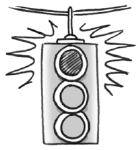### Home > CCA2 > Chapter 7 > Lesson 7.2.3 > Problem7-146

7-146.Colleen and Jolleen both used their calculators to find $\sin 30^\circ$. Colleen got $\sin 30^\circ=-0.9880316241$, but Jollen got $\sin 30^\circ=0.5$. Is one of their calculators broken, or is something else going on? Why did they get different answers?

Try calculating $\sin\left(30^\circ\right)$ in both degree mode and radian mode on your calculator.